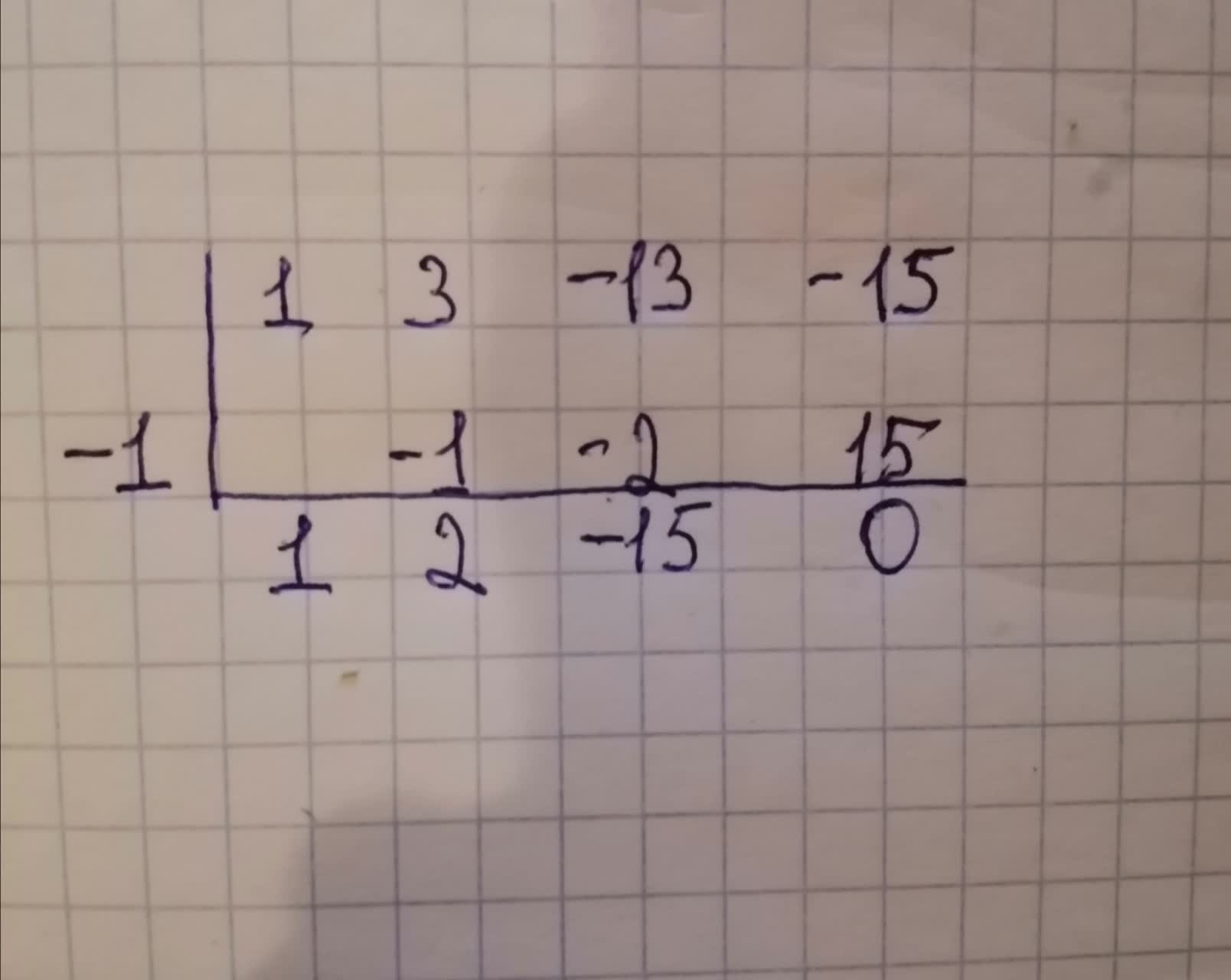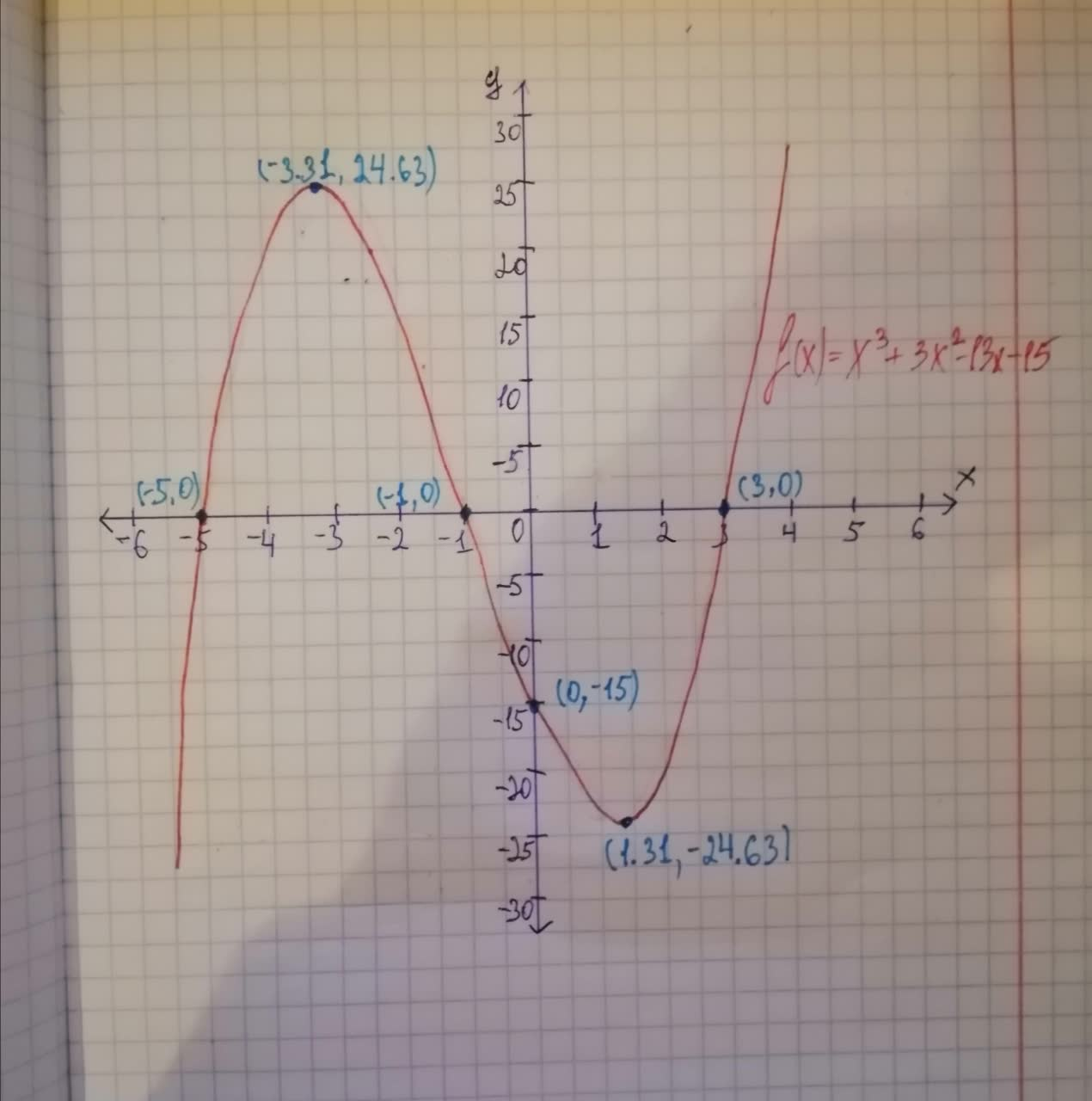# Graph each polynomial function. Factor first if the expression is not in factored form.f(x)=x^{3} + 3x^{2} - 13x - 15 PSZTobias Ali 2020-11-08 Answered

Graph each polynomial function. Factor first if the expression is not in factored form.

You can still ask an expert for help

• Questions are typically answered in as fast as 30 minutes

Solve your problem for the price of one coffee

• Math expert for every subject
• Pay only if we can solve itMacsen Nixon

The rational Root Theorem states that a rational root of a polynomial equation

q could equal any foctors of 1 So, Therefore, possible rational zeros are Since the polynomial is so, the cooefficient are 1, 3, -13, -15 Let 1 is an actual zero of the polynomial and use synthetic divisionThe remainder is 0 so, -1 is an actual zero and The equatient is

Factor the trinomial So, The function in factored form The function has three zeros -1, 3, and -5 So, the graph of f(x) crosses the x-axis at (-1, 0), (3, 0), and (-5, 0) To find the y-intercept, substitute 0 for x in f(x)
Substitute 0 for x So, the function f(x) crosses the y-axis at (0, -15) The leading coefficient is 1 Since the leading coefficient is positive and the function f(x) of degree 3 (odd degree) So, the end behavior is
See the graph below